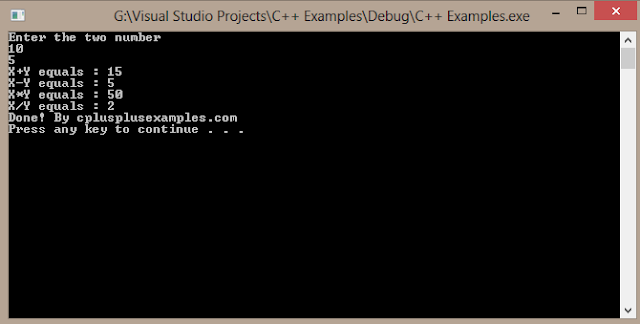### Basic C++ Operations & Examples

In any language, there are some operators to perform arithmetic, logical and control operations. The basic operators which are used to perform arithmetic operations on integers are as follows:

Operator                                    Operation

-                                                Subtraction, also called unary subtraction

*                                                Multiplication

/                                                Division

%                                               Modulus (remainder after division)

++                                             Increment

--                                               Decrements

The operators +,-,* and / perform the same operation as they do in other languages. The operators + and – are unary operators and can take one or two operands. The multiply and divide are called binary operators as they take two operands.

Integer division will always give the result which is of integer type and truncates the remainder. The output of integer division will be the quotient. For example, 5/2 will give the answer 2 and not 2.5. The modulus operator gives the remainder after the integer division. For example, 5/2 will give the answer 1, which is the remainder. Here is a program which illustrates the functioning of the operators.

Example C++ Source code :

#include
using namespace std;

int addition(int x,int y)
{
return(x+y);
}

int subtraction(int x,int y)
{
return(x-y);
}

int multiplication(int x,int y)
{
return(x*y);
}

int division(int x,int y)
{
return(x/y);
}

void main()
{
int x,y;
cout<<"Enter the two number"<
cin>>x;
cin>>y;

cout<<"X+Y equals : "<
cout<<"X-Y equals : "<<<
cout<<"X*Y equals : "<
cout<<"X/Y equals : "<
cout<<"Done! By cplusplusexamples.com"<
system("PAUSE");

}

Output of this program :

Enter the two number
55
5
X+Y equals : 60
X-Y equals : 50
X*Y equals : 275
X/Y equals : 11
Done! By cplusplusexamples.com
Press any key to continue . . .Cplusplusexamples.blogspot.com